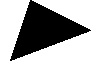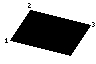# PLOTPL.

## Introduction

PLOT is a multi-purpose drawing statement. Typically three numbers follow the PLOT statement: the first specifies the type of point, line, triangle or circle to be drawn; the second and third give the X and Y coordinates to be used.

`PLOT Mode,X,Y`

Lines are drawn from the current graphics cursor position (the last point 'visited') to the specified (X,Y) coordinates. The graphics origin is usually the bottom-left corner of the screen, but it can be changed using the VDU 29 statement.

The two most commonly used statements, PLOT 4 and PLOT 5, have the duplicate keywords MOVE and DRAW.

## Plot Modes

Each drawing operation is assigned a numeric code. There are eight different numeric codes for each plotting mode, controlling the use of colour and whether the specified coordinates are absolute or relative to the last visited point.

Mode OffsetColourCoordinate
0NoneRelative
1Foreground
2Inverse
3Background
4NoneAbsolute
5Foreground
6Inverse
7Background

### Lines

BBC BASIC will draw a line between the two most recently visited points. For example,

```PLOT 4,10,20
PLOT 5,30,40```

draws a line in the current foreground colour between (10,20) and (30,40) inclusive. There are four different line patterns; solid, dotted, short dashes and long dashes.

Additionally, the last pixel of each line can be optionally drawn twice. This is most useful when drawing inverse-coloured lines, as it has the effect of not plotting the last pixel.

Mode RangeLine StyleLast Pixel Drawn Twice
0-7SolidNo
8-15Yes
16-23DottedNo
24-31Yes
32-39Short DashesNo
40-47Yes
48-55Long DashesNo
56-63Yes

### Points

Modes 64-71 will plot a single point, or pixel. You can retrieve the state of a point using the POINT function.

PLOT x,y is equivalent to PLOT 69,x,y. PLOT BY x,y is equivalent to PLOT 65,x,y.

### TrianglesModes 80-87 will plot and fill a triangle. The triangle is formed from the given X,Y coordinates and the previous two points 'visited', however they were specified.

The following example plots and fills the triangle shown with vertices at (30,0), (90,30) and (10,60) in the current graphics foreground colour.

```MOVE 30,0
MOVE 90,30
PLOT 85,10,60```

If the PLOT number is 81 or 85, the triangle is drawn and filled in the current foreground colour.

### Rectangles

Modes 96-103 will plot and fill an axis-aligned rectangle. The two most recently visited points are used for the two opposite corners of the rectangle.

### ParallelogramsModes 112-119 plot and fill a parallelogram whose vertices are defined by the previous two points visited and the point specified. For reference purposes, call the previous two points visited point1 and point2 in order, and the point specified in the PLOT command, point3. The x and y coordinates of the fourth point are calculated by point3-point2+point1.

The order in which the points are visited is important.

The following example plots and fills the parallelogram illustrated in the current graphics foreground colour.

```MOVE 10,40
MOVE 30,10
PLOT 117,90,25```

If the PLOT number is 113 or 117, the parallelogram will be drawn and filled in the current foreground colour.

### Flood Filling

Modes 128-135 and 136-143 can be used to flood fill a region of the screen starting from a given point, similar to the paint bucket tool found in image manipulation software.

• Modes 128-135 will start filling at the given point and continue until non-background pixels are found.
• Modes 136-142 will start filling at the given point and continue until foreground pixels are found.

For example, if you had a white region with a black border that you wished to fill you could either set the background colour to white and use one of modes 128-135 or set the foreground colour to black and use one of modes 136-142.

The following procedure acts like the paint bucket tool in an image manipulation program; it will allow you to fill regions of any colour with any other colour. It uses the POINT function to find the starting colour under the given point to set the correct background colour.

```DEF PROC_fill(x,y,c)
GCOL c
GCOL 128+POINT(x,y)
PLOT 133,x,y
ENDPROC```

Flood-filling is a memory-intensive operation, and if BBC BASIC is running low on memory it is possible for this plotting operation to trigger the untrappable 'No room' error.

### Circles

Modes 144-151 and 152-159 can be used to draw and fill circles respectively with the origin at the current graphics cursor position.

#### Point on Circumference

If the 'absolute' plot codes (148-151 or 156-159) are used the point specified will be on the circumference of the circle. For example,

```MOVE x1,y1
PLOT 149,x2,y2```

will draw a circle centred on (x1,y1) with the point (x2,y2) in its circumference.

If the 'relative' plot codes (144-147 or 152-155) are used either of the parameters may be used to specify the radius. For example, either

```MOVE x,y
PLOT 145,R,0```

or

```MOVE x,y
PLOT 145,0,R```

will draw a circle of radius R centred about the point (x,y).

### Ellipses

Modes 192-199 and 200-207 can be used to draw and fill ellipses respectively. Three points are required to draw an ellipse:

1. The origin (centre) of the ellipse.
2. A point on the vertical tangent of the ellipse.
3. A point on the horizontal tangent of the ellipse.

For example, to fill an ellipse centred around (x,y) with a horizontal radius hr and a vertical radius vr, you could use this code:

```MOVE x,y
PLOT 0,hr,0
PLOT 201,0,vr```

## Syntax

• PLOT <numeric>,<numeric>,<numeric>
• PLOT [BY] <numeric>,<numeric>